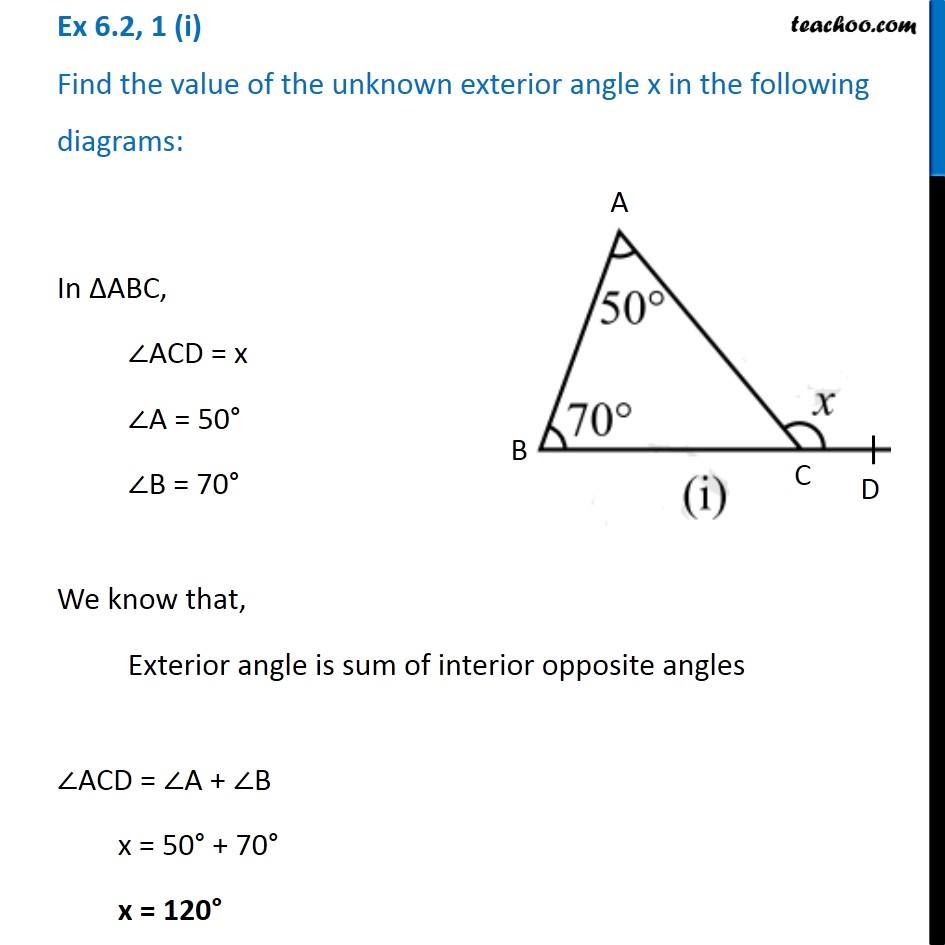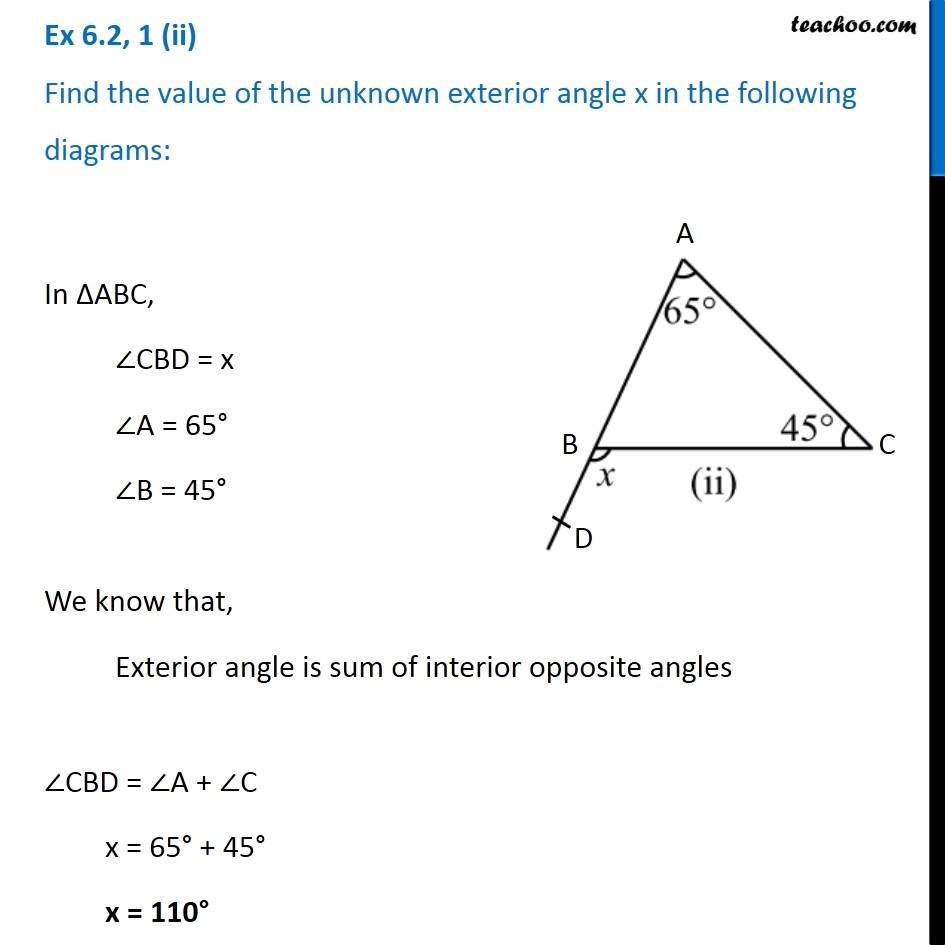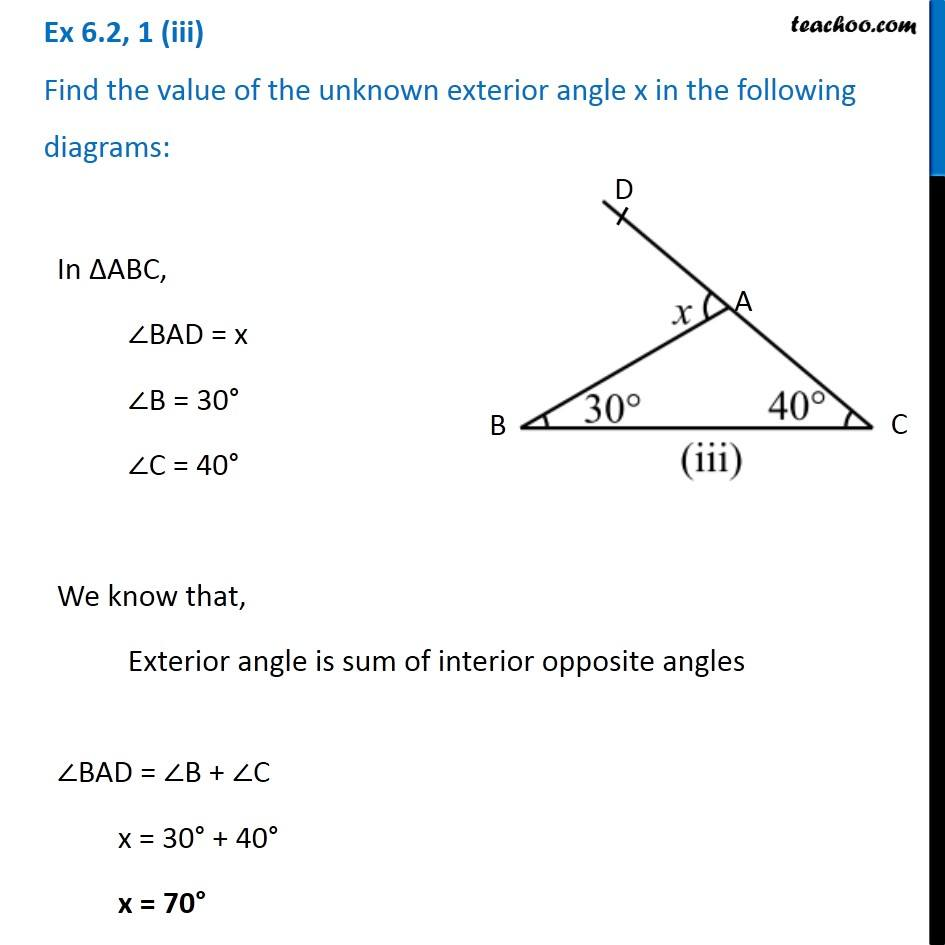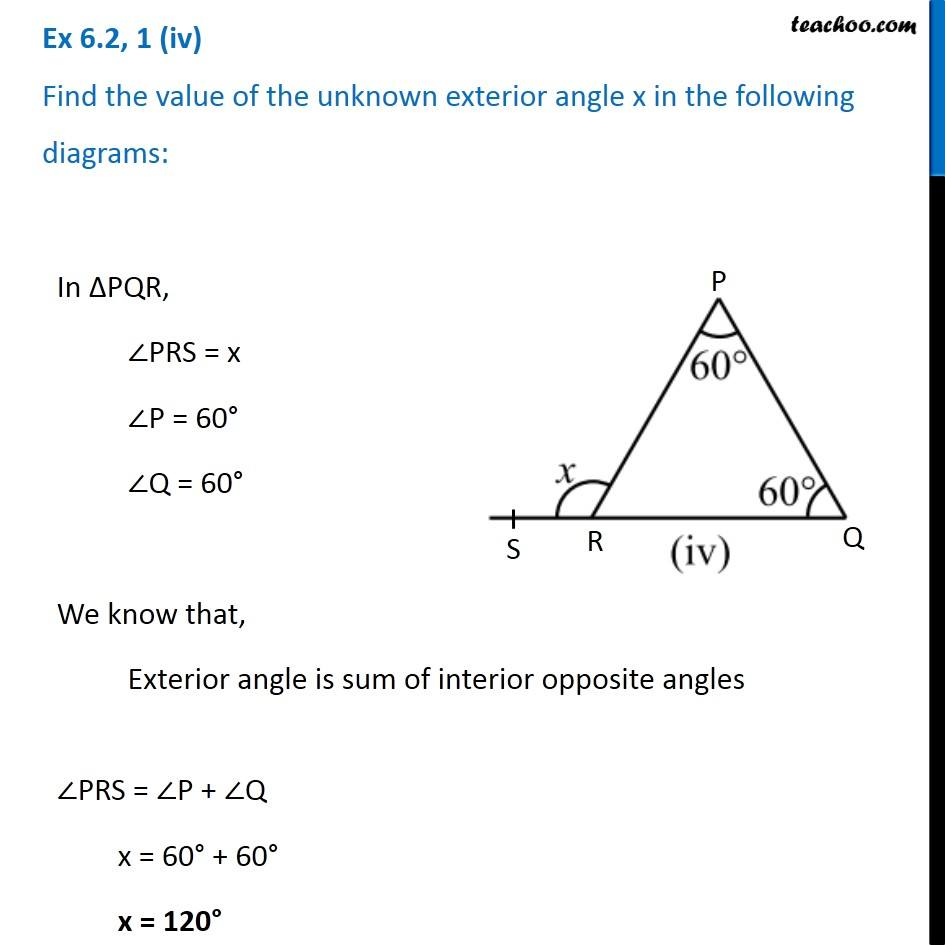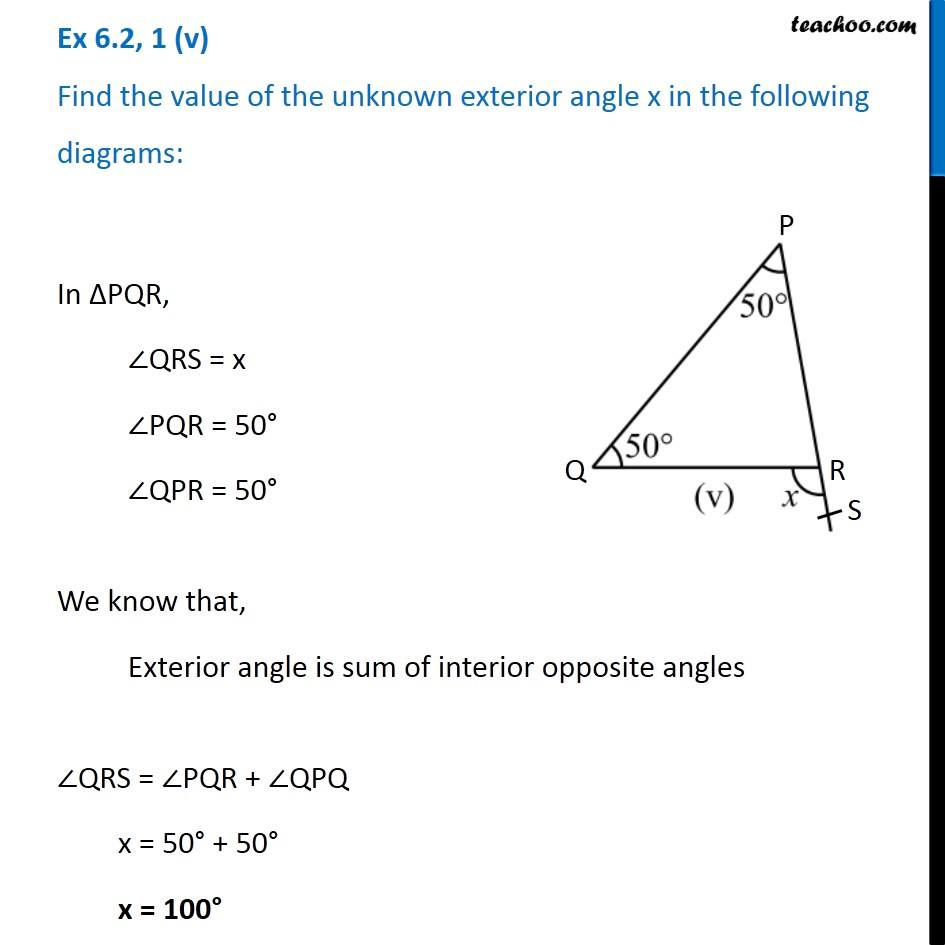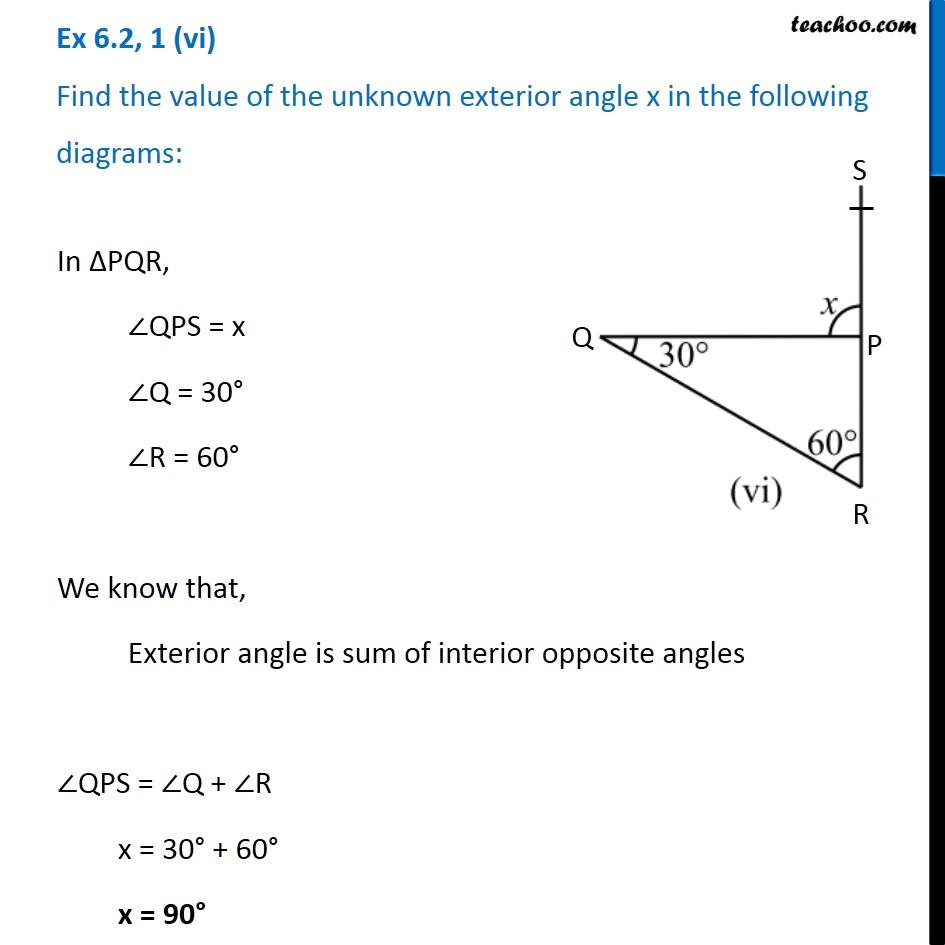Subscribe to our Youtube Channel - https://you.tube/teachoo

1. Chapter 6 Class 7 Triangle and its Properties
2. Serial order wise

Transcript

Ex 6.2, 1 (i) Find the value of the unknown exterior angle x in the following diagrams:In ∆ABC, ∠ACD = x ∠A = 50° ∠B = 70° We know that, Exterior angle is sum of interior opposite angles ∠ACD = ∠A + ∠B x = 50° + 70° x = 120° Ex 6.2, 1 (ii) Find the value of the unknown exterior angle x in the following diagrams:In ∆ABC, ∠CBD = x ∠A = 65° ∠B = 45° We know that, Exterior angle is sum of interior opposite angles ∠CBD = ∠A + ∠C x = 65° + 45° x = 110° Ex 6.2, 1 (iii) Find the value of the unknown exterior angle x in the following diagrams: In ∆ABC, ∠BAD = x ∠B = 30° ∠C = 40° We know that, Exterior angle is sum of interior opposite angles ∠BAD = ∠B + ∠C x = 30° + 40° x = 70° Ex 6.2, 1 (iv) Find the value of the unknown exterior angle x in the following diagrams:In ∆PQR, ∠PRS = x ∠P = 60° ∠Q = 60° We know that, Exterior angle is sum of interior opposite angles ∠PRS = ∠P + ∠Q x = 60° + 60° x = 120° Ex 6.2, 1 (v) Find the value of the unknown exterior angle x in the following diagrams:In ∆PQR, ∠QRS = x ∠PQR = 50° ∠QPR = 50° We know that, Exterior angle is sum of interior opposite angles ∠QRS = ∠PQR + ∠QPQ x = 50° + 50° x = 100° Ex 6.2, 1 (vi) Find the value of the unknown exterior angle x in the following diagrams:In ∆PQR, ∠QPS = x ∠Q = 30° ∠R = 60° We know that, Exterior angle is sum of interior opposite angles ∠QPS = ∠Q + ∠R x = 30° + 60° x = 90°